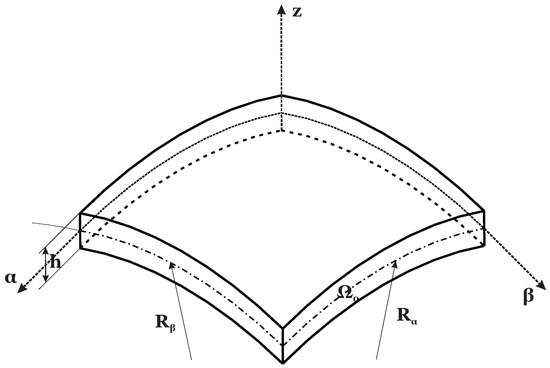Previous Article in Journal
Desert and Sonic Ecosystems: Incorporating Environmental Factors within Site-Responsive Sonic Art
Previous Article in Special Issue
Closed Form Solutions for Thermal Buckling of Functionally Graded Rectangular Thin Plates
Open AccessFeature PaperArticle

# Effects of Order of Expansion for the Exponential Matrix and Number of Mathematical Layers in the Exact 3D Static Analysis of Functionally Graded Plates and Shells

bySalvatore Brischetto *andRoberto TorreDepartment of Mechanical and Aerospace Engineering, Politecnico di Torino, corso Duca degli Abruzzi, 24, 10129 Torino, Italy
*
Author to whom correspondence should be addressed.
Appl. Sci. 2018, 8(1), 110; https://doi.org/10.3390/app8010110
Received: 22 December 2017 / Revised: 10 January 2018 / Accepted: 11 January 2018 / Published: 14 January 2018
This work deals with the study of the convergence ratio of the exponential matrix method used in the 3D static analysis of functionally graded structures subjected to harmonic loads. The equilibrium equations are written in mixed orthogonal curvilinear coordinates. This feature allows plates, cylinders, spherical and cylindrical shells to be studied with the same and unique formulation. After a reduction to first order differential equations, the obtained system is solved through the thickness by means of the exponential matrix method. The coefficients of these equations are not constant because the mechanical properties of the considered functionally graded materials change through the thickness. Moreover, the curvature terms introduce a further dependence of the coefficients from the thickness coordinate. The use of several mathematical layers allows for evaluating both the material properties and the curvature terms at certain points through the thickness direction. The M number of mathematical layers to be introduced is here studied in combination with the N expansion order of the exponential matrix. These values are influenced by several parameters. Therefore, different geometries, thickness ratios, lamination sequences, functionally graded material laws and half-wave numbers are considered. View Full-Text
Show FiguresFigure 1

MDPI and ACS Style

Brischetto, S.; Torre, R. Effects of Order of Expansion for the Exponential Matrix and Number of Mathematical Layers in the Exact 3D Static Analysis of Functionally Graded Plates and Shells. Appl. Sci. 2018, 8, 110.